International
Tables for
Crystallography
Volume A
Space-group symmetry
Edited by M. I. Aroyo

International Tables for Crystallography (2016). Vol. A, ch. 2.1, p. 162

## Section 2.1.3.11. Positions

Th. Hahna and A. Looijenga-Vosb

#### 2.1.3.11. Positions

| top | pdf |

The entries under Positions2 (more explicitly called Wyckoff positions) consist of the one General position (upper block) and the Special positions (blocks below). The columns in each block, from left to right, contain the following information for each Wyckoff position.

 (i) Multiplicity of the Wyckoff position. This is the number of equivalent points per unit cell. For primitive cells, the multiplicity of the general position is equal to the order of the point group of the space group; for centred cells, it is the product of the order of the point group and the number (2, 3 or 4) of lattice points per cell. The multiplicity of a special position is always a divisor of the multiplicity of the general position and the quotient of the two is equal to the order of the site-symmetry group. (ii) Wyckoff letter. This letter is merely a coding scheme for the Wyckoff positions, starting with a at the bottom position and continuing upwards in alphabetical order. (iii) Site symmetry. This is explained in Section 2.1.3.12. (iv) Coordinates. The sequence of coordinate triplets is produced in the same order as the symmetry operations, generated by the chosen set of generators, omitting duplicates (cf. Sections 1.4.3and 2.1.3.10). For centred space groups, the centring translations, for instance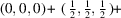, are listed above the coordinate triplets. The symbol' indicates that, in order to obtain a complete Wyckoff position, the components of these centring translations have to be added to the listed coordinate triplets. Note that not all points of a position always lie within the unit cell; some may be outside since the coordinates are formulated modulo 1; thus, for example,is written rather than. The coordinate triplets of a position represent the coordinates of the equivalent points (atoms) in the unit cell. A graphic representation of the points of the general position is provided by the general-position diagram; cf. Section 2.1.3.6. (v) Reflection conditions. These are described in Section 2.1.3.13.

Detailed treatment of general and special Wyckoff positions, including definitions, theoretical background and examples, is given in Section 1.4.4.

The two types of positions, general and special, are characterized as follows:

 (i) General position A point is said to be in general position if it is left invariant only by the identity operation but by no other symmetry operation of the space group. Each space group has only one general position. The coordinate triplets of a general position (which always start with x, y, z) can also be interpreted as a shorthand form of the matrix representation of the symmetry operations of the space group; this viewpoint is described further in Sections 1.3.3.2and 1.4.2.3. (ii) Special position(s) A point is said to be in special position' if it is mapped onto itself by the identity and at least one further symmetry operation of the space group. This implies that specific constraints are imposed on the coordinates of each point of a special position; e.g.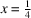,, leading to the triplet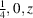; or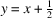, leading to the triplet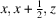. The number of special positions of a space group depends on the space-group type and can vary from 1 to 26 (in Pmmm, No. 47).

The set of all symmetry operations that map a point onto itself forms a group, known as the site-symmetry group' of that point. It is given in the third column by the oriented site-symmetry symbol' which is explained in Section 2.1.3.12. General positions always have site symmetry 1, whereas special positions have higher site symmetries, which can differ from one special position to another.

If in a crystal structure the centres of finite objects, such as molecules, are placed at the points of a special position, each such object must display a point symmetry that is at least as high as the site symmetry of the special position. Geometrically, this means that the centres of these objects are located on symmetry elements without translations (centre of symmetry, mirror plane, rotation axis, rotoinversion axis) or at the intersection of several symmetry elements of this kind (cf. the space-group diagrams).

Note that the location of an object on a screw axis or on a glide plane does not lead to an increase in the site symmetry and to a corresponding reduction of the multiplicity for that object. Accordingly, a space group that contains only symmetry elements with translation components does not have any special position. Such a space group is called `fixed-point-free' (for further discussion, see Section 1.4.4.2).

#### Example: Space group C12/c1 (15, unique axis b, cell choice 1)

The general position 8f of this space group contains eight equivalent points per cell, each with site symmetry 1. The coordinate triplets of four points, (1) to (4), are given explicitly, the coordinates of the other four points are obtained by adding the componentsof the C-centring translation to the coordinate triplets (1) to (4).

The space group has five special positions with Wyckoff letters a to e. The positions 4a to 4d require inversion symmetry,, whereas Wyckoff position 4e requires twofold rotation symmetry, 2, for any object in such a position. For position 4e, for instance, the four equivalent points have the coordinates. The values of x and z are specified, whereas y may take any value. Since each point of position 4e is mapped onto itself by a twofold rotation, the multiplicity of the position is reduced from 8 to 4, whereas the order of the site-symmetry group is increased from 1 to 2.

From the symmetry-element diagram of C2/c, the locations of the four twofold axes can be deduced as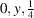;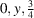;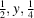;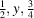.

From this example, the general rule is apparent that the product of the position multiplicity and the order of the corresponding site-symmetry group is constant for all Wyckoff positions of a given space group; it is the multiplicity of the general position.

Attention is drawn to ambiguities in the description of crystal structures in a few space groups, depending on whether the coordinate triplets of IT (1952)or of this edition are taken. This problem is analysed by Parthé et al. (1988).

### References

International Tables for X-ray Crystallography (1952). Vol. I, edited by N. F. M. Henry & K. Lonsdale. Birmingham: Kynoch Press. [Revised editions: 1965, 1969 and 1977. Abbreviated as IT (1952).]
Parthé, E., Gelato, L. M. & Chabot, B. (1988). Structure description ambiguity depending upon which edition of International Tables for (X-ray) Crystallography is used. Acta Cryst. A44, 999–1002.